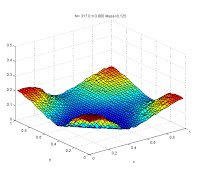### Hybrid multiscale methods for hyperbolic relaxation systems2D Burgers limit
Hyperbolic systems with relaxation arise in a wide variety of physical problems, ranging from linear and nonlinear waves, kinetic theory, to multiphase and phase transition modeling. We report here some numerical results of one-dimensional and two-dimensional computations using hybrid stochastic-deterministic methods of problems involving different scales.
The main components of the hybrid schemes is the use of probabilistic Monte Carlo methods for the full model (far from equilibrium regimes) combined with deterministic shock capturing techniques for the reduced one (close to equilibrium regimes). The prototype system used is the simple Jin-Xin model with relaxation limit given by the Burgers equations . The hybrid method is based on a stochastic simulation of the Jin-Xin dynamic (see ) combined with a second order finite volume relaxed scheme for the Burgers equation. The details of the method can be found in .  Subsequentely the method has been extended to kinetic equations and arbitrary fluid solvers in [3-4]. For one-dimensional results the stochastic component of the solution is depicted by red dots, the fluid component by a dashed blue line and the hybrid solution by green circles. In all computations the initial data is fully stochastic. See also my research activity [here] for further information.

1. Initial data sum of sines ε = 0.1.
2. 1. Initial data sum of sines ε = 0.01.

3. Two dimensional case. Inital data sine square ε = 0.01.

References
1. L.Pareschi, M.Seaid, A New Monte Carlo Approach for Conservation Laws and Relaxation Systems, Lecture Notes in Computer Science, Vol. 3037, pp:281-288 (2004)
2. G.Dimarco, L.Pareschi, Hybrid methods for multiscale problems I: hyperbolic relaxation system, Commun. Math. Sci. 4 (2006), no. 1, 155--177.
3. Dimarco, Giacomo ; Pareschi, Lorenzo . Hybrid multiscale methods. II. Kinetic equations. Multiscale Model. Simul. 6 (2007/08), no. 4, 1169--1197.
4. Dimarco G., Pareschi L. Fluid Solver Independent Hybrid Methods For Multiscale Kinetic Equations. Siam Journal On Scientific Computing, Vol. 32; P. 603-634, (2010).